# Sphere

Go to Surface Area or Volume.

### Sphere Facts

Notice these interesting things:• It is perfectly symmetrical
• All points on the surface are the
same distance "r" from the center
• It has no edges or
vertices (corners)
• It has one surface
(not a "face" as it isn't flat)
• It is not a polyhedron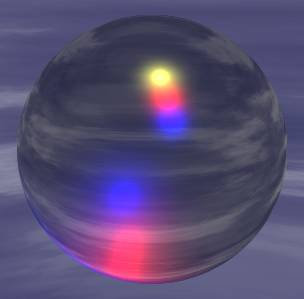Glass Sphere.
Balls and marbles are shaped like spheres.
images/balloon.js

## Largest Volume for Smallest Surface

Of all the shapes, a sphere has the smallest surface area for a volume. Or put another way it can contain the greatest volume for a fixed surface area.

Example: if you blow up a balloon it naturally forms a sphere because it is trying to hold as much air as possible with as small a surface as possible. Press the Play button to see.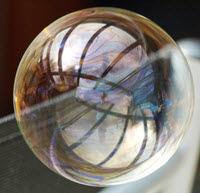## In Nature

The sphere appears in nature when a surface wants to be as small as possible. Examples include bubbles and water drops. Can you think of more?

## Volume and Surface AreaSurface Area = 4 × π × r2

### Example: r = 5

Surface Area = 4 × π × r2
= 4 × π × 52
= 4 × π × 25
= 100 π
≈ 314

Volume = (4/3) × π × r3

### Example: r = 5

Volume = (4/3) × π × r3
= (4/3) × π × 53
= (4/3) × π × 125
≈ 524

Try dragging point "A":

## Spheroid

### The Earth

The Planet Earth, our home, is nearly a sphere, except that it is squashed a little at the poles.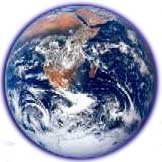So it is technically a spheroid, but a very mild version of one.

Here is a more extreme spheroid:## Hemisphere

A hemisphere is an exact half of a sphere.

## Other Cool Spheres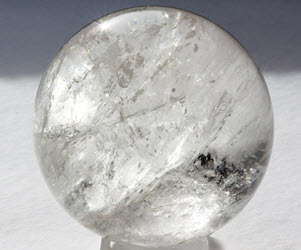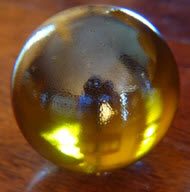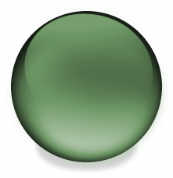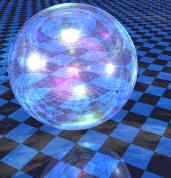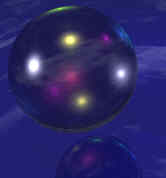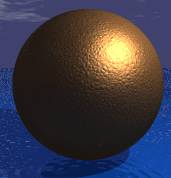865,866,867,868, 1831, 1832, 3383, 3384, 3385, 3386